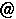English version
\$B@lLg!'0LAj4v2?3X!#(B
\$BFC\$K!"7k\$SL\\$H#3

\$B8&5f=82q!V(BIntelligence of Low-dimensional Topology\$B!W(B
(2022\$BG/(B5\$B7n(B25\$BF|!A(B27\$BF|!"5~ETBg3X(B \$B?tM}2r@O8&5f=j(B)

\$B8&5f=82q!V(BIntelligence of Low-dimensional Topology\$B!W(B
(2021\$BG/(B5\$B7n(B19\$BF|!A(B21\$BF|!"5~ETBg3X(B \$B?tM}2r@O8&5f=j(B)
• \$BL\$2r7hLdBj=8(B Problems on Low-dimensional Topology 2021 (pdf file)
\$B8&5f=82q!V(BIntelligence of Low-dimensional Topology\$B!W(B
(2020\$BG/(B5\$B7n(B13\$BF|!A(B15\$BF|!"5~ETBg3X(B \$B?tM}2r@O8&5f=j(B)
• \$BL\$2r7hLdBj=8(B Problems on Low-dimensional Topology 2020 (pdf file)
\$B9q:]2q5D!V(BEast Asian Conference on Geometric Topology\$B!W(B
(2020\$BG/(B2\$B7n(B10\$BF|!A(B13\$BF|!"5~ETBg3X(B \$B?tM}2r@O8&5f=j(B)

\$B8&5f=82q!V(BIntelligence of Low-dimensional Topology\$B!W(B
(2019\$BG/(B5\$B7n(B22\$BF|!A(B24\$BF|!"5~ETBg3X(B \$B?tM}2r@O8&5f=j(B)
• \$BL\$2r7hLdBj=8(B Problems on Low-dimensional Topology 2019 (pdf file)
\$B8&5f=82q!V(BIntelligence of Low-dimensional Topology\$B!W(B
(2018\$BG/(B5\$B7n(B30\$BF|!A(B6\$B7n(B1\$BF|!"5~ETBg3X(B \$B?tM}2r@O8&5f=j(B)
• \$BL\$2r7hLdBj=8(B Problems on Low-dimensional Topology 2018 (pdf file)
\$B8&5f=82q!V(BIntelligence of Low-dimensional Topology\$B!W(B
(2017\$BG/(B5\$B7n(B24\$BF|!A(B26\$BF|!"5~ETBg3X(B \$B?tM}2r@O8&5f=j(B)
• \$BL\$2r7hLdBj=8(B Problems on Low-dimensional Topology 2017 (pdf file)
\$B8&5f=82q!V(BIntelligence of Low-dimensional Topology\$B!W(B
(2016\$BG/(B5\$B7n(B18\$BF|!A(B20\$BF|!"5~ETBg3X(B \$B?tM}2r@O8&5f=j(B)
• \$BL\$2r7hLdBj=8(B Problems on Low-dimensional Topology 2016 (pdf file)

\$BBh(B62\$B2s%H%]%m%8!<%7%s%]%8%&%`(B
(2015\$BG/(B8\$B7n(B6\$BF|!A(B9\$BF|!"L>8E209)6HBg3X(B)

2015\$BG/(B6\$B7n(B20\$BF|(B N-KOOK\$B%;%_%J!<(B \$B<+M39V1i(B
\$BBgDPCNCi!V7k\$SL\\$N(BKontsevich\$BITJQNL\$H(BMilnor move\$B!W(B \$B9V1i;qNA(B

\$B8&5f=82q!V(BIntelligence of Low-dimensional Topology\$B!W(B
(2015\$BG/(B5\$B7n(B20\$BF|!A(B22\$BF|!"5~ETBg3X(B \$B?tM}2r@O8&5f=j(B)
• \$BL\$2r7hLdBj=8(B Problems on Low-dimensional Topology 2015 (pdf file)
• \$B8&5f=82q\$NJs9p=8\$,(B \$B?tM}8&9V5fO?(B1960 \$B\$H\$7\$F=PHG\$5\$l\$F\$\$\$^\$9!#(B
\$B8&5f=82q!VB?MMBN\$N%H%]%m%8!<\$NE8K>!W(B
(2014\$BG/(B11\$B7n(B28\$B!A(B30\$BF|!"El5~Bg3X(B \$B?tM}2J3X8&5f2J(B)

\$BBh(B61\$B2s%H%]%m%8!<%7%s%]%8%&%`(B
(2014\$BG/(B7\$B7n(B26\$BF|!A(B29\$BF|!"ElKLBg3X(B)

\$B8&5f=82q!V(BIntelligence of Low-dimensional Topology\$B!W(B
(2014\$BG/(B5\$B7n(B21\$BF|!A(B23\$BF|!"5~ETBg3X(B \$B?tM}2r@O8&5f=j(B)
• \$BL\$2r7hLdBj=8(B Problems on Low-dimensional Topology 2014 (pdf file)
• \$B8&5f=82q\$NJs9p=8\$,(B \$B?tM}8&9V5fO?(B1911 \$B\$H\$7\$F=PHG\$5\$l\$F\$\$\$^\$9!#(B
\$B8&5f=82q!V(BIntelligence of Low-dimensional Topology\$B!W(B
(2013\$BG/(B5\$B7n(B22\$BF|!A(B24\$BF|!"5~ETBg3X(B \$B?tM}2r@O8&5f=j(B)
• \$BL\$2r7hLdBj=8(B Problems on Low-dimensional Topology 2013 (pdf file)
• \$B8&5f=82q\$NJs9p=8\$,(B \$B?tM}8&9V5fO?(B1866 \$B\$H\$7\$F=PHG\$5\$l\$F\$\$\$^\$9!#(B
\$B8&5f=82q!V(BIntelligence of Low-dimensional Topology\$B!W(B
(2012\$BG/(B5\$B7n(B16\$BF|!A(B18\$BF|!"5~ETBg3X(B \$B?tM}2r@O8&5f=j(B)
• \$BL\$2r7hLdBj=8(B Problems on Low-dimensional Topology 2012 (pdf file)
• \$B8&5f=82q\$NJs9p=8\$,(B \$B?tM}8&9V5fO?(B1812 \$B\$H\$7\$F=PHG\$5\$l\$F\$\$\$^\$9!#(B
\$B8&5f=82q!V(BIntelligence of Low-dimensional Topology\$B!W(B
(2011\$BG/(B5\$B7n(B25\$BF|!A(B27\$BF|!"5~ETBg3X(B \$B?tM}2r@O8&5f=j(B)
• \$BL\$2r7hLdBj=8(B Problems on Low-dimensional Topology 2011 (pdf file)
• \$B8&5f=82q\$NJs9p=8\$,(B \$B?tM}8&9V5fO?(B1766 \$B\$H\$7\$F=PHG\$5\$l\$F\$\$\$^\$9!#(B
\$B8&5f=82q!V(BIntelligence of Low-dimensional Topology\$B!W(B
(2010\$BG/(B6\$B7n(B2\$BF|!A(B4\$BF|!"5~ETBg3X(B \$B?tM}2r@O8&5f=j(B)
• \$BL\$2r7hLdBj=8(B Problems on Low-dimensional Topology (pdf file)
• \$B8&5f=82q\$NJs9p=8\$,(B \$B?tM}8&9V5fO?(B1716 \$B\$H\$7\$F=PHG\$5\$l\$F\$\$\$^\$9!#(B
2008\$BG/(B8\$B7n(B6\$BF|(B(\$B?e(B)\$B\$N(B \$B%H%]%m%8!<%7%s%]%8%&%`\$G\$N9V1i(B
\$BBgDPCNCi!V%Y%C%A?t\$,(B1\$B\$N(B3\$B \$B\$NM=9F=8869F(B
ps file (1.8 M bytes), pdf file (0.3 M bytes)

Sergei Duzhin \$B;a\$K\$h\$kO"B39V5A!V(BDetecting the link orientation\$B!W(B
\$B!J(B2006\$BG/(B9\$B7n(B28\$BF|!"(B29\$BF|!K(B
tomotadakurims.kyoto-u.ac.jp